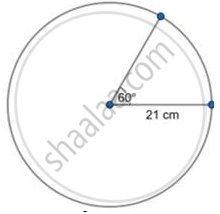# Find the Area of Sector Whose Central Angle and Radius Are 60o and 21 Cm Respectively. (Pi = 22/7) - Geometry

Diagram

Find the area of sector whose central angle and radius are 60o and 21 cm respectively.
(pi = 22/7)

#### SolutionArea =theta/360 xx pi"r"^2

⇒ A = 60/360 xx 22/7 xx 21^2

⇒ A = 1/6 xx 22/7 xx 21 xx 21

⇒ A = 11 × 21

⇒ A = 231 cm2

Concept: Tangent to a Circle
Is there an error in this question or solution?